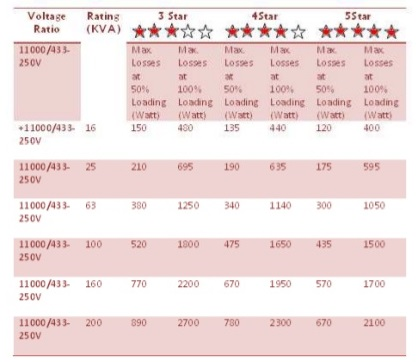# How to Measures Core and Winding Losses of Electrical Current Transformer

Both short circuit and open circuit tests are conducted to measure the core and winding losses of transformers. As we know that there are mainly two major parts of transformers i.e. core and winding, which are made of two type of materials, which are iron and copper.  The core of a transformer is made of iron whereas the winding which is wrapped over the iron core is made up of copper.

Therefore based upon the types of the materials there are two types of transformer losses i.e. iron losses and copper losses. In order to measure the copper losses short circuit test is conducted. This test is also known as full load test, copper loss test and winding loss test.

On the other hand to measure the iron losses open circuit test is conducted. This test is also known as no load test, iron loss test or core loss test. Now we shall learn more details about both the transformer loss measuring or testing procedures.

## Short Circuit or Full Load Test of Electrical Transformer

The short circuit test useful for measuring the winding losses of a current transformer and is conducted on the high voltage HV side of the transformer. In this procedure the secondary winding (usually Low Voltage Side) of the transformer is short circuited. After that full load current is applied on the primary side of the transformer.

Since the core losses are very small and can be neglected because applied voltage is very small as compared to the nominal voltage. Therefore the watt meter measures only full load copper losses.

The following picture shows the arrangement for conducting Short Circuit Test using DPATT-3Bi. You can see that LV side is short circuited and the voltage is applied at HV side.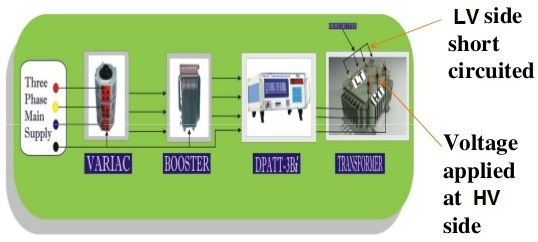### Steps for conducting Short Circuit Test in Electrical Current Transformer

Step 1 – Find Full Load Current: Calculate Full Load Current from parameters available in Transformer’s name plate as described below. The values can be taken from name plate of the electrical current transformer rating:

HT Voltage = 11KV

LT Voltage = 415V (P-P)

KVA Rating = 200 KVA

Full Load Current Formula = KVA Rating x 1000 / √3 x HV Line Voltage = 200 x1000 / √3 x 11000

Full Load Current = 10.49 A

The value of Full Load Current for Transformers of different ratings is given below for reference.

 Rating of Transformer Full Load Current 200KVA 10.48A 300KVA 15.72A 400KVA 20.96A 500KVA 26.2A 600KVA 31.44A

Step 2 – Short Circuiting: Now short circuit LT side and apply the required voltage at HT side of electrical current transformer until the full load current is achieved.

Step 3 – Calculations: Now calculate Impedance Voltage (the voltage at which full load current is achieved) and then calculate % Impedance as per the following formula.

% Impedance = (Impedance Voltage / Rated Voltage) * 100

Suppose the Full Load Current, 10.49A is obtained at 463.1V therefore the Impedance Voltage is 463.1V.

% Impedance = (Impedance Voltage / Rated Voltage) * 100 = (463.1 * 100) / 11000 = 4.21

On Pressing F2 button of the keypad of DPATT-3Bi instrument, the parameters of Short Circuit Test are displayed on the LCD as shown below. This is a big advantage of DPATT-3Bi that it can be used for Transformer Testing.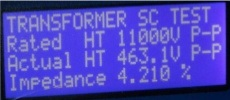On pressing button ‘3’ of the keypad of DPATT-3Bi, W/PF page appears on the LCD Display showing Copper Losses.

## Open Circuit or No Load Transformer Testing

As stated earlier on this page the open circuit test is carried out to determine iron losses in electrical current transformers. We all know that transformers dissipate electrical energy whenever they are On. Core losses and magnetizing current depends upon the applied voltage and frequency. Since we have no control on variation of mains frequency therefore the applied voltage is varied to keep V/f ratio constant. Always the core is designed in a way for minimizing the core losses.

The following picture shows the arrangement for conducting core loss open circuit test using DPATT-3Bi instrument. You can observe that voltage is applied at LT side and HT side is having open circuit.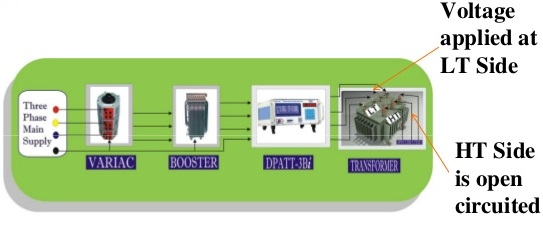### Steps for Open Circuit Test to Measure Transformer Core Losses

Step 1: Obtain the value of Rated Voltage from parameters given in transformer’s Name Plate as explained below.

Example: According to transformer’s name plate

HT Voltage = 11KV

LT Voltage = 415V (P-P)

KVA Rating = 200 KVA

Rated Voltage = 11KV (Primary Side)

Rated Voltage = 415V (Secondary Side)

Step 2: Open circuit HT Side while apply voltage at LT Side through Variac until the Rated Voltage is achieved. It is very important to note that if supply frequency is different from rated frequency, the voltage to be applied is calculated as per supply frequency.

Illustration:

Rated LT Voltage VRT = 415 V

Rated Frequency FRT = 50 Hz

If, Supply frequency FS =49.50Hz

Voltage to be applied, VA = (VRT / FRT ) * FS VA = (415 / 50) * 49.50 = 410.85V

On Pressing F2 button of the keypad of DPATT-3Bi, the parameters of short circuit test are displayed on the LCD Display as shown below.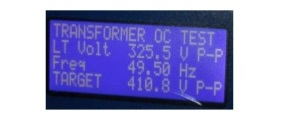This is a big advantage of DPATT-3Bi that it can be used for transformer Testing.

Step 3: Now you can calculate no load current as described below.

Illustration:

Transformer Rating

HT Voltage= 11KV ,

Rated Voltage = 415V

LT Voltage=415V ,

Rating of T/F= 200KVA

According to BEE

No Load Current = 2.5% of Full Load Current = (10.49* 2.5 / 100)